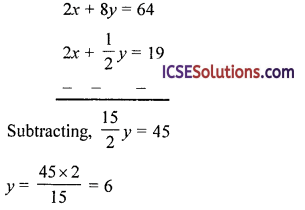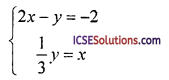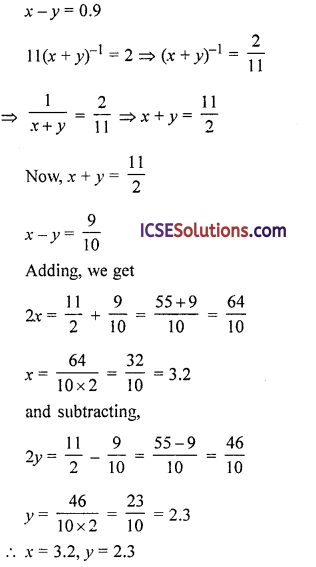Students appreciate clear and concise OP Malhotra Class 9 Solutions Chapter 5 Simultaneous Linear Equations in Two Variables Chapter Test that guide them through exercises.

## S Chand Class 9 ICSE Maths Solutions Chapter 5 Simultaneous Linear Equations in Two Variables Chapter Test

Solve :

Question 1.
y + 2x = 5 3y – 5x = 4
Solution:
y + 2x = 5 ⇒ y = 5 – 2x
3y – 5x = 4
⇒ 3(5 – 2x) – 5x = 4
⇒ 15 – 6x – 5x = 4
⇒ – 11x = 4 – 15
⇒ – 11x = – 11
and y = 5 – 2x = 5 – 2 x 1 = 5 – 2 = 3
∴ x = 1, y = 3

Question 2.
$$\frac { 1 }{ 2 }$$x + 2y = 16
2x + $$\frac { 1 }{ 2 }$$y = 19
Solution:
$$\frac { 1 }{ 2 }$$x + 2y = 16 … (i)
2x + $$\frac { 1 }{ 2 }$$y = 19 … (ii)
Multiply (i) by 4 and (ii) by 1From (i) $$\frac { 1 }{ 2 }$$x + 2 x 6 = 16 ⇒ $$\frac { 1 }{ 2 }$$x = 16 – 12
⇒ $$\frac { 1 }{ 2 }$$x = 4 ⇒ x = 4 x 2 = 8
∴ x = 8, y = 6Question 3.
Which ordered pair is a solution of the system?(a) (0, 2)
(b) (2, 6)
(c) (1, 3)
(d) (3, 8)
Solution:
(b) (2, 6)
2x – y = – 2, $$\frac { 1 }{ 3 }$$y = x ⇒ y = 3x
⇒ 2x – 3x = – 2 ⇒ – x = – 2 ⇒ x = 2
and y = 3x = 3 x 2 = 6
∴ x = 2, y = 6
∴ Order pair of solution is (2, 6)

Question 4.
Which of the following problems could be solved by finding the solution of the given system?
2x + 2y = 56, y = $$\frac { 1 }{ 3 }$$x
(a) The area of a reactangle is 56 sq. unit. The width is one-third the length. Find the length of the rectangle.
(b) The area of a rectangle is 56 sq. unit. The length is one-third the perimeter. Find the length of the rectangle.
(c) The perimeter of a rectangle is 56 unit. The length is one-third more than the width. Find the length of the rectangle.
(d) The perimeter of a rectangle is 56 unit. The width is one-third the length. Find the length of the rectangle.
Solution:
(d) The perimeter of a rectangle is 56 unit. The width is one-third the length. Find the length of the rectangle.
2x + 2y = 56, y = $$\frac { 1 }{ 3 }$$x
Here 2x + 2y = 56 ⇒ 2(x + y) = 56
i.e., perimeter of a rectangle whose length
and breadth x and y is 56
and breadth = $$\frac { 1 }{ 3 }$$ length
∴ It is applies to (d)
Perimeter of rectangle = 56 unit
2(l + b) = 56
As breadth is one third of length
∴ 2(x + $$\frac { x }{ 3 }$$) = 56
= $$\frac{4 x}{3}=\frac{56}{2} \Rightarrow x=\frac{56}{2} \times \frac{3}{4}$$ = 21 unit

Question 5.
What is the solution of the equation x – 7 = 0.9 and 11 (x + y)-1 = 2?
(a) x = 3.2, y = 2.3
(b) x = 1, y = 0.1
(c) x = 2, y = 1.1
(d) x= 1.2, y = 0.3
Solution:
(a) x = 3.2, y = 2.3Question 6.
A number consists of two digits, whose sum is 10. If 18 is subtracted from the number, digits of the number are reversed. What is the product of the digits?
(a) 15
(b) 18
(c) 24
(d) 32
Solution:
(c) 24
Let unit digit of a two digit number = x
and ten digit = y
∴ x + 7 = 10 … (i)
and number = x + 10y
By reversing the digits, the number = y + 10x
∴ x + 10y – 18 = y + 10x
⇒ x + 10y – y – 10x = 18
– 9x + 97 = 18
– 9(x – 7)= 18 ⇒ x – y = $$\frac { 18 }{ -9 }$$ = – 2
Now, x + y = 10
x – y = – 2
2x = 8 ⇒ x = $$\frac { 8 }{ 2 }$$ = 4
Subtracting,
27 = 12 ⇒ y = $$\frac { 12 }{ 2 }$$ = 6
∴ Product of digits = x × y = 4 x 6 = 24Question 7.
If $$\frac{2 x-3 y+1}{2}=\frac{x+4 y+8}{3}=\frac{4 x-7 y+2}{5}$$, then what is (x + 7) equal to?
(a) 3
(b) 2
(c) 0
(d) – 2
Solution:
(d) – 2
$$\frac{2 x-3 y+1}{2}=\frac{x+4 y+8}{3}=\frac{4 x-7 y+2}{5}$$
$$\frac{2 x-3 y+1}{2}=\frac{x+4 y+8}{3}$$
⇒ 6x – 97 + 3 = 2x + 87 + 16
⇒ 6x – 97 – 2x – 87 = 16 – 3
⇒ 4x – 17y = 13
$$\frac{x+4 y+8}{3}=\frac{4 x-7 y+2}{5}$$
5x + 20y + 40 = 12x – 217 + 6
5x + 20y – 12x + 217 = 6 – 40
– 7x + 41y = – 34
7x – 41y = 3y … (i)
4x – 17y = 13 … (ii)
Multiply (i) by 4 and (ii) by
28x – 164y = 136
28x – 119y = 91
–         +          –
Subtracting, we get
– 45y = 45
y = $$\frac { 45 }{ -45 }$$ = – 1
From (ii),
4x – 17 x (- 1) = 13
⇒ 4x + 17 = 13 ⇒ 4x = 13 – 17 = – 4
⇒ x = $$\frac { -4 }{ 4 }$$ = – 1
∴ x + y = – 1 +(- 1) = – 1 – 1 = – 2

Question 8.
A railway ticket for a child costs half the full fare but the reservation charge is the same on half tickets as much as on full ticket. One reserved first class full ticket for a journey between two stations is ₹ 362; one full and one half reserved first class tickets cost ₹ 554. What is the reservation charge?
(a) ₹18
(b) ₹ 22
(c) ₹ 38
(d) ₹ 46
Solution:
(b) ₹ 22
Let reservation charges = ₹ x per ticket
and let price of full ticket = ₹ y
and half ticket = ₹$$\frac { 1 }{ 2 }$$
Now according to the question,
x + y = ₹ 362 … (i)
2x + y + $$\frac { y }{ 2 }$$ = ₹ 554
⇒ 2x + $$\frac { 3 }{ 2 }$$y = ₹ 554 … (ii)
Multiply (i) by $$\frac { 3 }{ 2 }$$ and (ii) by 1
$$\frac{3}{2} x+\frac{3}{2} y=362 \times \frac{3}{2}=543$$
2x + $$\frac { 3 }{ 2 }$$y = 554
Subtracting (i) from (ii),
$$\frac { 1 }{ 2 }$$x = 11 ⇒ x = 11 x 2 = 22
Cost of reservation = ₹ 22

Question 9.
What is the sum of two numbers whose difference is 45 and the quotient of the greater number by the lesser number is 4?
(a) 100
(b) 90
(c) 80
(d) 75
Solution:
(d) 75
Let first number = x
and second number = y
∴ x – y = 45 … (i)
$$\frac { x }{ y }$$ = 4 ⇒ x = 4y … (ii)
From (i) 4y – y = 45
⇒ 3y = 45
y = $$\frac { 45 }{ 3 }$$ = 15
∴ x = 4 x 15 = 60
First number = 60
and second number = 15
Sum of two number = x + y
= 60 + 15 = 75Question 10.
Two numbers are in the ratio 2 : 3. If 19 is added to each number, they will be in the ratio 3 : 4. What is the product of the two numbers?
(a) 360
(b) 480
(c) 486
(d) 512
Solution:
(c) 486
Ratio in two number = 2 : 3
Let first number = x
and second number = y
Then, $$\frac { 1 }{ 2 }$$ = $$\frac { 1 }{ 2 }$$ ⇒ 3x = 2y
⇒ x = $$\frac { 2 }{ 3 }$$y … (i)
Adding 9 to each, we get
x + 9 and y + 9
∴ $$\frac{x+9}{y+9}=\frac{3}{4}$$ ⇒ 4x + 36 = 3y + 27
= 4x – 3y = 27 – 36 = – 9 … (i)
⇒ 4 x $$\frac { 2 }{ 3 }$$y – 3y = – 9 [From (i)]
⇒ $$\frac { 8 }{ 3 }$$y – 3y = – 9
$$\frac{8 y-9 y}{3}$$ = – 9 ⇒ $$\frac { -y }{ 3 }$$ = – 9
⇒ y = – 9 x (- 3) = 27
and x = $$\frac { 2 }{ 3 }$$ x 27 = 18
∴ Numbers are 18, 27
Product of two numbers = 18 x 27
= 486